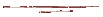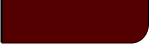Projects Lab

Course MaterialInstructor Hub# Online Learning MaterialTony Butterfield, University of Utah

print

Statistics, Basics:

• Basics of Statistics - An introduction to means, standard deviation, population vs. sample properties, and so on.

• Random Variables, PDFs, and CDFs - Basic information on random variables and their associated functions.

• Display of Data - An brief discussion of ways in which data is typically displayed, with interactive examples.

• Useful Statistics Functions - a collection of basic statistics functions and plots thereof, which are important in the realm of data analysis.

Data Analysis:

Physical Measurements:

Experimental Design:

Online Tools:

• Analysis of a single data set (one treatment) - Finds basic statistical properties, calculates confidence intervals at a given level, and tests normality.

• Analysis of two data sets (two treatment) - Finds basic statistical properties, calculates confidence intervals at a given level, performs hypothesis tests, and tests normality.

• Error Propagation - Given a function, the value of its variables and their error, this script approximates the propagated error in the calculated value (discussed in greater detail here).

• Parabolic PDE Example - Example of numerical solutions to the 2D transient diffusion problem under various boundary conditions. Parabolic PDEs may describe a variety of phenomena, from heat and mass transfer to acoustic propagation and EM field strength.

Heat and Mass Transfer:

• Parabolic PDE Example - Example of finite difference solutions to the 2D transient diffusion problem under various boundary conditions. Parabolic PDEs may describe a variety of phenomena, from heat and mass transfer to acoustic propagation and EM field strength.